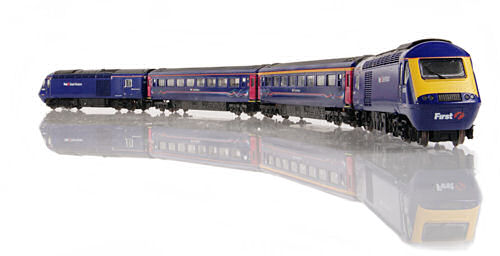# Scale SpeedJOHN CHANDLER explains how to calculate the correct scale speed for your locomotives.

See more GENERAL MODELLING

## Introduction/Bête Noire

One of the things that irks me, both at Club and Exhibition level is the speed at which the trains are run.

Normally, this is just based on the judgement of the operator at the time and may be wildly inaccurate, but there may be a more scientific approach.

Normal trains run at around 100 to 130mph. Japanese bullet trains on some lines can reach 180mph. The SNCF TGV routinely runs at 200mph. As no commercial models are yet available of maglev trains, I'll overlook them for this article.

So how does this relate to the model situation? I beg your tolerance as I present both a spreadsheet for the common speeds and also the formulae on which it is based so that you can calculate for differing scales and speeds.

I appreciate that devices can now be purchased which display both the model's own speed on the layout and its full scale equivalent speed. However, these fall outside of my remit.

## What is Speed?

Speed is, in general, the rate at which a train (sorry, I'm concerned mainly with trains here, but the principle equally applies to any other kinds of model machine) either travels from point to point or passes a specific location (for example: the place from where you are watching the train).

Measured in either miles per hour (mph) or kilometres per hour (Kmh), it represents the distance travelled by the train in a given time period. In other words, a train travelling at a constant speed of 100mph will travel 100 miles in one hour!

## What is Scale?

Models of full sized trains are built to be a scale replica. Being of simple mind, I'll use what has become known as Continental N Scale for my examples; this uses a scale ratio of 1:160. This means that every yard of the full scale locomotive is represented by approximately 0.2 inches on the model. (1 yard = 36 inches, 36/160 = 0.225 inches).

My favourite (and somewhat paradoxical) take on this is that: 1 mile = 1760 yards; in Continental N Scale of 1:160, dividing 1760 yards by the scale ratio of 160 results in exactly 11.0 yards (a non-continental unit of measurement)! Check it on your calculator. (Using British N Scale at 1:148, 1 mile is represented by 11.891891891891891... yards of track!)

On the other hand, time does not scale; one minute at full scale is as long as one minute at 1:160 scale. I'm awaiting the barrage from those operators who run their layout to a timetable! That's a totally different kettle of fish and possible subject for a future article!

Given, therefore, that unless Albert Einstein is in the vicinity, time remains constant at whatever scale we are working, the distance travelled by the train in a certain time can easily be determined and measured.

## Calculation

To determine that a train model is running at an appropriate speed, and without using any commercially available speed monitors, it is necessary to measure a length of easily accessible (to the operators) length of track; the longer the better.

As the train enters the measured section, a stopwatch is started; as it leaves the section, the said stopwatch is stopped. The elapsed time is recorded.

Let's put some mathematical constants down here:

1 mile = 1760 yards (= 5280 feet)

1 hour = 60 minutes = 3600 seconds

We're working here at Continental N Scale of 1:160.

So, let's say that our full scale train is travelling at 160mph.

Converting to Continental N Scale, 160 miles reduces to 160/160 = 1 mile. So our model should be travelling at 1mph.

Let's get a more measurable handle on this; timing a train for one hour to check that it has travelled one mile is slightly impractical; so let's go to a more manageable time frame.

As stated, one hour equates to 3600 seconds. Thus, in one second, our model should have travelled 1/3600th of a mile, i.e. 17.6 inches.

Let's put the boot on the other foot; I have a measured distance (say, one yard) on the layout, how long should the train take to traverse it?

We've already established that a full scale train travelling at 160mph should, in our scale world, travel one mile in one hour, or 17.6 inches in one second, so what is a practical way of checking this? Let's time our model over one yard.

1 yard = 36 inches = 36 / 17.6 seconds = approximately 2 seconds.

So, to represent a full scale train travelling at 160mph in Continental N Scale, it should travel one yard of track in approximately 2 seconds.Is your HST going too fast or too slow?

Using the attached spreadsheet, it is possible to determine the speed at which your model should be travelling to represent a scale speed of its prototype. Note that some scale speeds may be impractical to achieve, for example: a G Scale (implicitly narrow gauge) is unlikely to exceed 60mph; however, these are calculated in the spreadsheet for completeness! (0 Scale TGVs do exist!) You can amend the information in the orange cells and the spreadsheet will calculate the correct Yards Per Second and Seconds Per Yard figure.

It should also be noted that, for example: the scale of H0 implicitly includes all derivatives, such as H0e and H0m. Simply, this table is independent of gauge. A static version of the spreadsheet is shown below.Click image to view larger version.

## Conclusion

Rather than guess the realistic speed at which a model should be run, it is possible to calculate a more precise speed at which to fully represent the operation of its prototype.